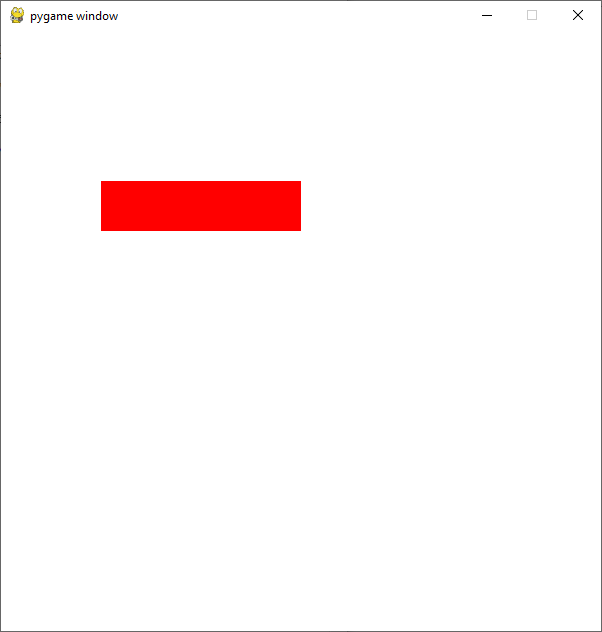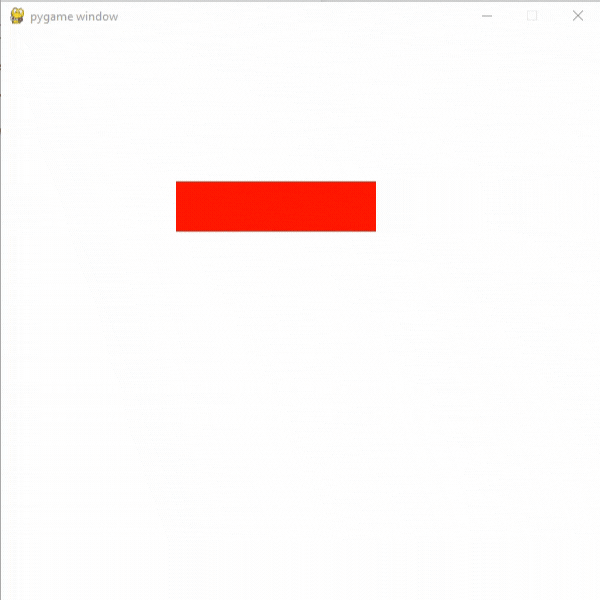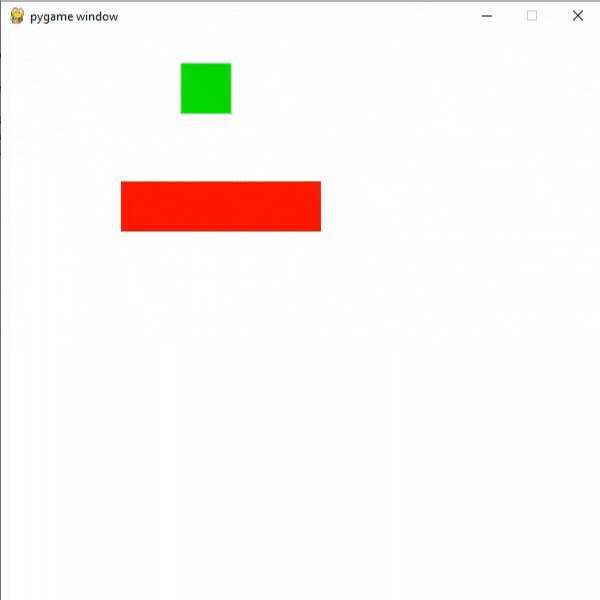# How to add moving platforms in PyGame

• Difficulty Level : Expert
• Last Updated : 18 Oct, 2021

Prerequisite: Drawing in Pygame

In this article, we will learn how we can add moving platforms to our game using PyGame in Python.

Attention geek! Strengthen your foundations with the Python Programming Foundation Course and learn the basics.

To begin with, your interview preparations Enhance your Data Structures concepts with the Python DS Course. And to begin with your Machine Learning Journey, join the Machine Learning - Basic Level Course

## Creating a Platform

We can easily create any type of platform in pygame using the draw( ) method. For this, we will create react with specific width and height using the draw.rect() function.

Syntax: pygame.draw.rect(surface, color, rect, width)

Parameters:

• surface : Here we can pass the surface on which we want to draw our rectangle.
• color : Here we can pass the color for our rectangle.
• rect : Here we can pass the rectangle, position, and dimensions.
• width : Here we can pass the line thickness. we can also create a solid rectangle by changing the value of this width parameter.

Code:

## Python3

 `# Importing the pygame module``import` `pygame``from` `pygame.``locals` `import` `*` `# Initiate pygame and give permission``# to use pygame's functionality``pygame.init()` `# Create a display surface object``# of specific dimension``window ``=` `pygame.display.set_mode((``600``,``600``))` `# Creating a new clock object to``# track the amount of time``clock ``=` `pygame.time.Clock()` `# Starting coordinates of the platform``x ``=` `100``y ``=` `150` `# Creating a rect with width``# and height``rect ``=` `Rect(x, y, ``200``, ``50``)` `# Creating a boolean variable that``# we will use to run the while loop``run ``=` `True` `# Creating an infinite loop``# to run our game``while` `run:` `    ``# Setting the framerate to 30fps``    ``clock.tick(``30``)` `    ``# Drawing the rect on the screen using the``    ``# draw.rect() method``    ``pygame.draw.rect(window, (``255``, ``0``, ``0``),rect)``    ` `    ``# Updating the display surface``    ``pygame.display.update()` `    ``# Filling the window with white color``    ``window.fill((``255``,``255``,``255``))`

Output:## Moving the Platform

To move the platform we can create a velocity variable with some numeric value and we can add that velocity to the x coordinate of our platform. After that, we will multiply the velocity variable with -1 if its x coordinate is less than 100 or greater than or equal to 300.

Code:

## Python3

 `# Importing the pygame module``import` `pygame``from` `pygame.``locals` `import` `*` `# Initiate pygame and give permission``# to use pygame's functionality``pygame.init()` `# Create a display surface object``# of specific dimension``window ``=` `pygame.display.set_mode((``600``,``600``))`  `# Creating a new clock object to``# track the amount of time``clock ``=` `pygame.time.Clock()` `# Variable to store the``# velocity of the platform``platform_vel ``=` `5` `# Starting coordinates of the platform``x ``=` `100``y ``=` `150` `# Creating a rect with width``# and height``rect ``=` `Rect(x, y, ``200``, ``50``)` `# Creating a boolean variable that``# we will use to run the while loop``run ``=` `True`  `# Creating an infinite loop``# to run our game``while` `run:` `    ``# Setting the framerate to 30fps``    ``clock.tick(``30``)` `    ``# Multiplying platform_vel with -1``    ``# if its x coordinate is less then 100``    ``# or greater than or equal to 300.``    ``if` `rect.left >``=``300` `or` `rect.left<``100``:``        ``platform_vel``*``=` `-``1` `    ``# Adding platform_vel to x``    ``# coordinate of our rect``    ``rect.left ``+``=` `platform_vel` `    ``# Drawing the rect on the screen using the``    ``# draw.rect() method``    ``pygame.draw.rect(window, (``255``,   ``0``,   ``0``),rect)` `    ``# Updating the display surface``    ``pygame.display.update()` `    ``# Filling the window with white color``    ``window.fill((``255``,``255``,``255``))`

Output:## Adding Player Sprite and Collision

Now we are going to add our player and collision between our player and platform. For this, we are using colliderect() method.

Syntax: pygame.Rect.colliderect(rect1 , rect2)

Parameters: It will take two rects as its parameters.

Returns true if any portion of either rectangle overlap.

If the player is colliding with the platform then we will set the coordinate of player bottom equal to the top of the platform and then we will add the platform velocity. We are also creating a gravity variable.

Code:

## Python3

 `# Importing the pygame module``import` `pygame``from` `pygame.``locals` `import` `*` `# Initiate pygame and give permission``# to use pygame's functionality``pygame.init()` `# Create a display surface object``# of specific dimension``window ``=` `pygame.display.set_mode((``600``,``600``))` `# Creating a new clock object to``# track the amount of time``clock ``=` `pygame.time.Clock()` `# Variable to store the``# velocity of the platform``platform_vel ``=` `5` `# Starting coordinates of the platform``x ``=` `100``y ``=` `150` `# Starting coordinates for``# player sprite``player_x ``=` `180``player_y ``=` `0` `# Creating a new variable``# for gravity``gravity ``=` `8` `# Creating a new rect for player``player_rect ``=` `Rect(player_x, player_y, ``50``, ``50``)` `# Creating a rect with width``# and height``rect ``=` `Rect(x, y, ``200``, ``50``)` `# Creating a boolean variable that``# we will use to run the while loop``run ``=` `True` `# Creating an infinite loop``# to run our game``while` `run:` `    ``# Setting the framerate to 30fps``    ``clock.tick(``30``)` `    ``# Multiplying platform_vel with -1``    ``# if its x coordinate is less then 100``    ``# or greater than or equal to 300.``    ``if` `rect.left >``=``300` `or` `rect.left<``100``:``        ``platform_vel``*``=` `-``1` `    ``# Checking if player is colliding``    ``# with platform or not using the``    ``# colliderect() method.``    ``# It will return a boolean value``    ``collide ``=` `pygame.Rect.colliderect(rect, player_rect)` `    ``# If player is colliding with``    ``# platform then setting coordinate``    ``# of player bottom equal to top of platform``    ``# and adding the platform velocity``    ``if` `collide:``        ``player_rect.bottom ``=` `rect.top``        ``player_rect.left ``+``=` `platform_vel` `    ``# Adding platform_vel to x``    ``# coordinate of our rect``    ``rect.left ``+``=` `platform_vel` `    ``# Adding gravity``    ``player_rect.top ``+``=` `gravity` `    ``# Drawing the rect on the screen using the``    ``# draw.rect() method``    ``pygame.draw.rect(window, (``255``,   ``0``,   ``0``),rect)` `    ``# Drawing player rect``    ``pygame.draw.rect(window, (``0``,   ``255``,   ``0``),player_rect)` `    ``# Updating the display surface``    ``pygame.display.update()` `    ``# Filling the window with white color``    ``window.fill((``255``,``255``,``255``))`

Output:My Personal Notes arrow_drop_up